Intermediate Geometry : Spheres

Example Questions

1 2 3 4 5 6 7 9 Next →

Example Question #4 : How To Find The Radius Of A Sphere

If the volume of a sphere is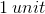, what is the sphere's exact radius?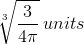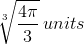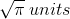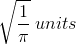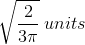Explanation:

Write the formula for the volume of a sphere: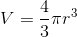Plug in the given volume and solve for the radius,.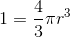Start by multiplying each side of the equation by: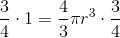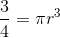Now, divide each side of the equation by: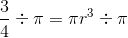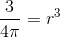Finally, take the cubed root of each side of the equation: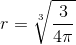Example Question #5 : How To Find The Radius Of A Sphere

Given the volume of a sphere is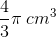, what is the radius?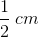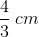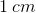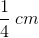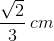Explanation:

The equation for the volume of a sphere is:, whereis the length of the sphere's radius.

Plug in the given volume and solve forto calculate the sphere's radius: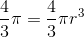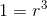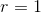Example Question #6 : How To Find The Radius Of A Sphere

If the volume of a sphere is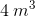, what is the radius of the sphere?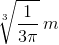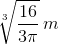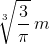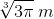Explanation:

The formula for the volume of a sphere is:, whereis the sphere's radius.

Plug in the volume and solve for, the sphere's radius: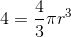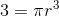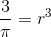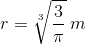Example Question #7 : How To Find The Radius Of A Sphere

Find the radius of a sphere if the surface area is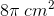.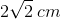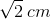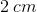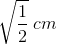Explanation:

The formula for the surface area of a sphere is: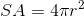Substitute the given value for the sphere's surface area into the equation and solve forto find the radius: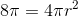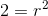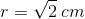Example Question #8 : How To Find The Radius Of A Sphere

Find the radius of a sphere if its surface area is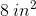.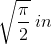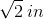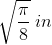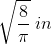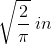Explanation:

The surface area formula for a sphere is: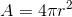, whereis the sphere's radius.

Substitute the given value for the sphere's area into the equation and solve forto find the radius: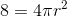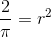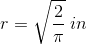1 2 3 4 5 6 7 9 Next →